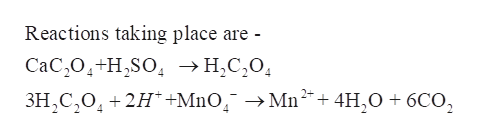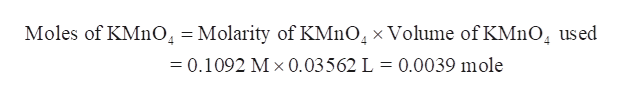# A 1.2516 gram sample of a mixture of CaCO3 and Na2SO4 was analyzed by dissolving the sample and completely precipitating the Ca2+ as CaC2O4. The CaC2O4 was dissolved in sulfuric acid and the resulting H2C2O4 was titrated with a standard KMnO4 solution. The titration required 35.62 mL of 0.1092 M KMnO4. Calculate the number of moles of H2C2O4 that reacted with the KMnO4. Calculate the number of moles of CaCO3 in the original sample. Calculate the percentage by weight of CaCO3 in the original sample.

Question
93 views

A 1.2516 gram sample of a mixture of CaCO3 and Na2SO4 was analyzed by dissolving the sample and completely precipitating the Ca2+ as CaC2O4. The CaC2O4 was dissolved in sulfuric acid and the resulting H2C2Owas titrated with a standard KMnOsolution. The titration required 35.62 mL of 0.1092 M KMnO4. Calculate the number of moles of H2C2Othat reacted with the KMnO4. Calculate the number of moles of CaCO3 in the original sample. Calculate the percentage by weight of CaCO3 in the original sample.

check_circle

Step 1

A 1.2516 gram sample of a mixture of CaCO3 and Na2SO4 was analysed by dissolving the sample and completely precipitating the Ca2+ as CaC2O4. The CaC2O4 was dissolved in sulfuric acid and the resulting H2C2Owas titrated with a standard KMnOsolution. The titration required 35.62 mL of 0.1092 M KMnO4. Calculate the number of moles of H2C2Othat reacted with the KMnO4. The number of moles of CaCO3 in the original sample is to be calculated. The percentage by weight of CaCO3 in the original sample is to be determined.

Step 2

From the mixture, CaCO3 is precipitated out as CaC2O4. Thus1 mole of CaC2O4 is precipitated by 1 mole of CaCO3. And this 1 mole of CaC2O4 is converted into 1 mole of H2C2O4.

Further H2C2O4 is titrated with KMnO4.help_outlineImage TranscriptioncloseReactions taking place are - СаC,0,+H,SO, > Н,С,0, ЗН,С, О, +2H +MnO, > Mn** + 4H,0 + 6C0, fullscreen
Step 3

Calculate the moles of KMnO4 reacted:

Molarity of KMnO4 = 0.1092

Volume of KMnO4...help_outlineImage TranscriptioncloseMoles of KMnO = Molarity of KM1O4 x Volume of KMnO, used =0.1092 M x 0.03 5 62 L = 0.0039 mole fullscreen

### Want to see the full answer?

See Solution

#### Want to see this answer and more?

Solutions are written by subject experts who are available 24/7. Questions are typically answered within 1 hour.*

See Solution
*Response times may vary by subject and question.
Tagged in

### Inorganic Chemistry